21.2 (EAP/Beta)
21.1
20.2
20.1
19.2
19.1
The page you are viewing does not exist in version 19.1. This link will take you to the root page.
18.2
The page you are viewing does not exist in version 18.2. This link will take you to the root page.
18.1
The page you are viewing does not exist in version 18.1. This link will take you to the root page.
17.2
The page you are viewing does not exist in version 17.2. This link will take you to the root page.

# HeatmapPoint.Value Property

Gets or sets the heatmap point value.

Namespace: DevExpress.XtraMap

Assembly: DevExpress.XtraMap.v21.1.dll

## Declaration

``````[DefaultValue(0)]
public double Value { get; set; }``````

## Property Value

Type Default Description
Double

0

The heatmap point value.

## Remarks

Points with higher values have more saturated colors.

The Map Control uses the following algorithm to color heatmap points. If the Value property is not specified, it is set to 0.4. Pixel weight (value) is calculated for all pixels inside the point radius. The Map Control uses the following formula: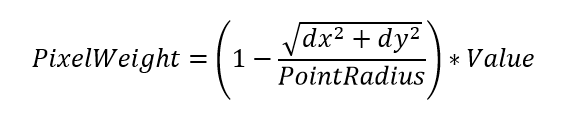where:

• dx/dy - the distance from the heatmap point center in pixels along the X and Y axes, respectively;
• Value - HeatmapPoint.Value (the heatmap point value).

For example, the point radius is 10. The heatmap point’s central pixel has a weight that equals the point value. The weight of the pixel located next to the central pixel (dx = 1, dy = 0) is 0.9*Value, the second pixel from the center (dx = 2, dy = 0) is 0.8*Value, etc.

Central Pixel
PointRadius =10; Point Value = 2
Pixel Value = 2
Third pixel from the center
PointRadius =10; Point Value = 2
Pixel Value = (1 - 3/10)*2= 1.4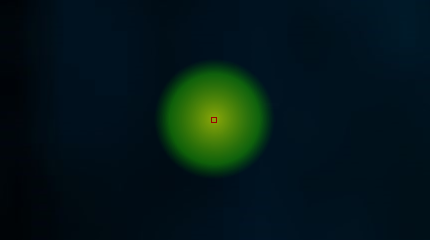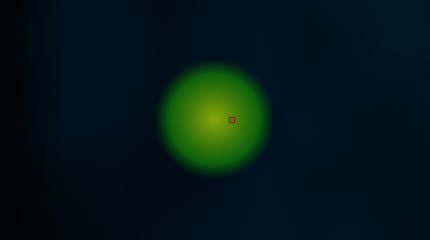The Map Control summarizes values from different heatmap points for each pixel. Since the point radius is set in pixels, the Map Control reaggregates heatmap points when you zoom in to or zoom out of a map. For this reason, areas with aggregated points can change colors.

In the right image, heatmap points are closer to each other. Pixels between them are included in the point radiuses of two heatmap points. The Map Control calculates values for these pixels and colors them according to these values.

Zoom Level = 5 Zoom Level = 4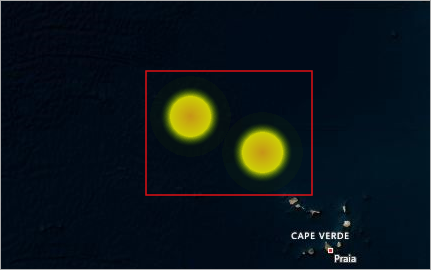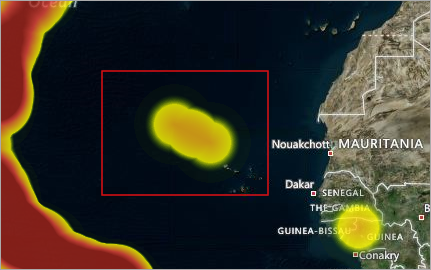The Map Control uses the Colorizer to color pixels based on their values. The colorizer gets colors from the ColorItems collection to paint pixels with values from the RangeStops collection. Pixels with values between range stops are colored with mixed colors. If you do not define the colorizer, the Map Control uses the following colorizer code:

``````ColorCollection colors = new ColorCollection {
Color.FromArgb(10, 0, 128, 0),
Color.FromArgb(200, 25, 153, 0),
Colors.Yellow,
Colors.Red
};
ChoroplethColorizer colorizer = new ChoroplethColorizer {
ApproximateColors = true,
Colors = colors,
RangeStops = new DoubleCollection(new double[] { 0, 0.1, 0.5, 1 })
};
``````
##### NOTE

When you do not define Colorizer and set the same value for all points (or do not specify point values), the Map Control sets point values to 0.4. This approach allows the default colorizer to work correctly. When the default colorizer is used, the Map Control displays the legend with a scale from 0 to 1.

## Example

This example shows how to create a heatmap layer for the Map Control.

``````using DevExpress.XtraMap;
using System;
using System.Collections.Generic;
using System.Data;
using System.Drawing;
using System.Windows.Forms;

namespace HeatMapSample {
public partial class Form1 : Form {
public Form1() {
InitializeComponent();
}
private void Form1_Load(object sender, EventArgs e) {

ChoroplethColorizer colorizer = new ChoroplethColorizer();
colorizer.RangeStops.AddRange(new double[] { 0.1, 0.2, 0.7, 1 });
colorizer.ApproximateColors = true;

HeatmapPointStorage pointStorage = new HeatmapPointStorage();
new HeatmapPoint(new GeoPoint(23.5309, -0.4211), 1),
new HeatmapPoint(new GeoPoint(32.3248, 21.0537), 0.5),
new HeatmapPoint(new GeoPoint(14.1503, 16.3626), 1),
new HeatmapPoint(new GeoPoint(7.2144,  34.2711), 1),
new HeatmapPoint(new GeoPoint(-4.5456, 10.1143), 0.5)
});

HeatmapProvider provider = new HeatmapProvider();
provider.PointSource = pointStorage;
provider.Algorithm = new HeatmapDensityBasedAlgorithm { PointRadius = 50 };
provider.Colorizer = colorizer;

ImageLayer heatmapLayer = new ImageLayer();
heatmapLayer.DataProvider = provider;